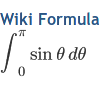## Plugins Directory » Wiki GChart Formula Plugin

Author: Website:Masamitsu Murase https://github.com/masamitsu-murase/redmine_wiki_gchart_formula https://github.com/masamitsu-murase/redmine_wiki_gchart_formula.git 2011-08-23 (almost 10 years ago) 0.0.5 Redmine 1.2.x, 1.3.x (0)

# wiki_gchart_formula (LaTeX-style formula plugin for Redmine)¶

## Overview¶

• This Redmine plugin enables Redmine to render LaTeX-style formula images in Wiki.
• This plugin uses Google Chart API, so it works without any other software, such as latex or dvips.
• You can use curly braces in formula.

## How to write in Redmine Wiki¶

You can write formula in LaTeX style as follows:

{{latex(formula)}}

For example,

{{latex(x=\frac{-b\pm\sqrt{b^2-4ac}}{2a})}}

is shown as.

formula can include curly braces, '{' and '}'.

If you need more information about formula, please refer to Google Chart API and LaTeX commands.

## Samples¶

• {{latex(\textstyle\sum_{k=1}^n k^2)}} is shown as.
• {{latex(\displaystyle\sum_{k=1}^n k^2)}} is shown as.
• {{latex(\displaystyle\sum_{k=1}^n k^3 = \left(\frac{1}{2}n(n+1)\right)^2)}} is shown as.
• {{latex(\displaystyle A=\left(\begin{matrix} 1 & 2 & 3 \\ 4 & 5 & 6 \\ 7 & 8 & 9 \end{matrix}\right))}} is shown as.

## Tips¶

• This plugin regards the sequence ')}}' as an end of the LaTeX formula, so you should write ') }}' instead of ')}}' to output ')}}'.
• Too long formula cannot be shown, because of Google Chart API restriction.
If you need to output long formula, you should consider using another plugin, such as redmine-wiki_latex_plugin.
• This plugin uses Google Chart API, so the formula you input is sent to Google.
If you are nervous about the privacy, you should use another plugin instead of this plugin.
• You can use curly braces in formula, such as {{latex(\sum_{k=1}^n k^2)}}, because this plugin does not use Redmine::WikiFormatting::Macros but has own processing method, inline_wiki_gchart_formula.
If you would like to know more, please refer to wiki_gchart_formula_patch.rb.
• class attribute, "gchart_formula", is added to the image tag of formula.

## License¶

You can use this plugin under the MIT License.
This plugin uses Google Chart API, so you have to obey Terms of Service of Google Chart Tools.

### Installation notes

1. Extract "redmine_wiki_gchart_formula.zip" into #{RAILS_ROOT}/vendor/plugins.
2. Restart Redmine.
Migration is not needed.

### Changelog

#### 0.0.5 (2012-01-26)

Compatible with Redmine 1.2.x, 1.3.x.

Bug fix about conflicts with some plugins.
Thank you for your report, Ryan Edwards!

#### 0.0.4 (2012-01-22)

Compatible with Redmine 1.2.x, 1.3.x.

Bug fix about 'ampersand' error.
Thank you for your report, Yoav HaCohen!

#### 0.0.2 (2011-08-23)

Compatible with Redmine 1.0.x, 1.1.x, 1.2.x, 1.3.x.